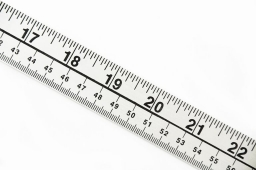# Determine 7836

We want to cut a 90 cm long rod into two parts so that the longer part of the rod is 2 cm shorter than three times the shorter part. Determine the lengths of both parts of the rod.

a =  67 cm
b =  23 cm

### Step-by-step explanation:

a+b=90
a = 3b-2

a+b=90
a = 3·b-2

a+b = 90
a-3b = -2

Row 2 - Row 1 → Row 2
a+b = 90
-4b = -92

b = -92/-4 = 23
a = 90-b = 90-23 = 67

a = 67
b = 23

Our linear equations calculator calculates it.Did you find an error or inaccuracy? Feel free to write us. Thank you!

Tips for related online calculators
Do you have a linear equation or system of equations and looking for its solution? Or do you have a quadratic equation?
Do you want to convert length units?